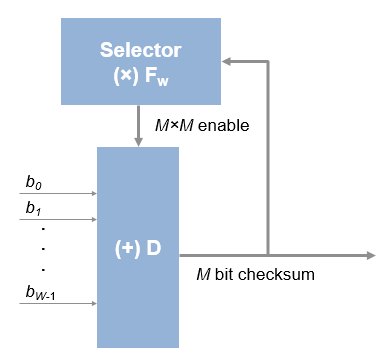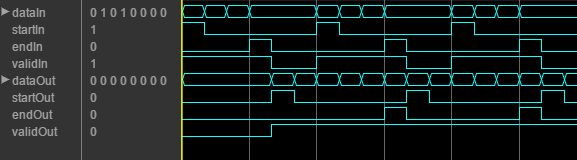Documentation

# General CRC Generator HDL Optimized

Generate CRC code bits and append to input data, optimized for HDL code generation

## Library

Communications Toolbox > Error Correction and Detection > CRC (`commcrc2`)

Communications Toolbox HDL Support > Error Correction and Detection > CRC (`commhdlcrc`)## Description

This hardware-friendly CRC generator block, like the General CRC Generator block, generates a cyclic redundancy check (CRC) checksum and appends it to the input message. With the General CRC Generator HDL Optimized block, the processing is optimized for HDL code generation. Instead of processing an entire frame at once, the block accepts and returns a data sample stream with accompanying control signals. The control signals indicate the validity of the samples and the boundaries of the frame. To achieve higher throughput, the block accepts vector data up to the CRC length, and implements a parallel architecture.

### Signal Attributes

PortDirectionDescriptionData Type
`dataIn`InputMessage data. Data can be a vector of binary values, or a scalar integer representing several bits. For example, vector input [0 0 0 1 0 0 1 1] is equivalent to uint8 input 19. The data width must be less than or equal to the CRC length, and the CRC length must be divisible by the data width. For example, for CRC-CCITT/CRC-16, the valid data widths are 16, 8, 4, 2, and 1.

Vector: double or Boolean

Scalar: unsigned integer (uint8/16/32) or fixdt(0,N,0)

`startIn`InputIndicates the start of a frame of data. Boolean
`endIn`InputIndicates the end of a frame of data. Boolean
`validIn`InputIndicates that input data is valid. Boolean
`dataOut`OutputMessage data with the checksum appended. The output data has the same vector size as the input data.Same as `dataIn`
`startOut`OutputIndicates the start of a frame of data. Boolean
`endOut`OutputIndicates the end of a frame of data, including checksum. Boolean
`validOut`OutputIndicates that output data is valid. Boolean

## Parameters

Polynomial

A double or Boolean vector specifying the polynomial, in descending order of powers. CRC length is length(polynomial) – 1. The default value is [1 0 0 0 1 0 0 0 0 0 0 1 0 0 0 0 1].

Initial state

A double or Boolean scalar or vector of length equal to the CRC length, specifying the initial state of the internal shift register. The default value is 0.

Direct method
• When this parameter is selected, the block uses the direct algorithm for CRC checksum calculations.

• When this parameter is not selected, the block uses the nondirect algorithm for CRC checksum calculations.

The parameter is cleared by default.

To learn about the direct and non-direct algorithms, see Cyclic Redundancy Check Codes.

Reflect input

The input data width must be a multiple of 8.

• When this parameter is selected, each input byte is flipped before entering the shift register.

• When this parameter is not selected, the message data is passed to the shift register unchanged.

The parameter is cleared by default.

Reflect CRC checksum

The CRC length must be a multiple of 8.

• When this parameter is selected, each checksum byte is flipped before it is passed to the final XOR stage.

• When this parameter is not selected, the checksum byte is passed to the final XOR stage unchanged.

The parameter is cleared by default.

Final XOR value

The value that the CRC checksum is XORed with before it is appended to the input data. This parameter can be a double or Boolean scalar or vector of length equal to the CRC length. The default value is 0.

## Algorithm

When you use vector or integer input, the block implements a parallel CRC algorithm .

To provide high throughput for modern communications systems, the CRC algorithm is implemented with a parallel architecture. This architecture recursively calculates M bits of CRC checksum for each W input bits. At the end of the frame, the final checksum result is appended to the message. For a polynomial length of M, the recursive checksum calculation for W bits in parallel is:

`${X}^{\text{'}}={F}_{W}\left(×\right)X\left(+\right)D$`

FW is an M-by-M matrix that selects elements of the current state for the polynomial calculation with the new input bits. D is an M-sample vector that provides the new input bits, ordered in relation to the polynomial and padded with zeroes. (×) is implemented with logical AND, and (+) is implemented with logical XOR.### Timing Diagram

This waveform shows streaming data and the accompanying control signals for a CRC16 with 8-bit binary vector input. There must be enough space between the input frames to insert the checksum word.This waveform diagram shows continuous input data. Non-continuous data is also supported. The output valid signal matches the input valid pattern.

### Initial Delay

The General CRC Generator HDL Optimized block introduces a latency on the output. This latency can be computed with the following equation, assuming the input data is continuous:

`initialdelay = (CRC length/input data width) + 2`

 Campobello, Giuseppe, Giuseppe Patane, and Marco Russo. "Parallel CRC Realization." IEEE Transactions on Computers. Vol. 52, No. 10, October 2003, pp. 1312–1319.